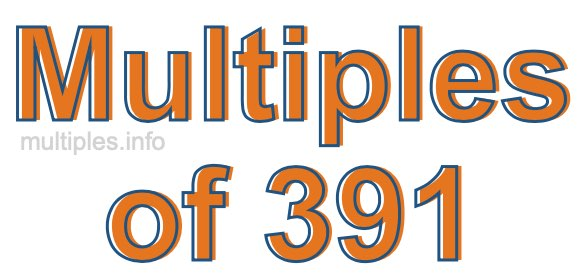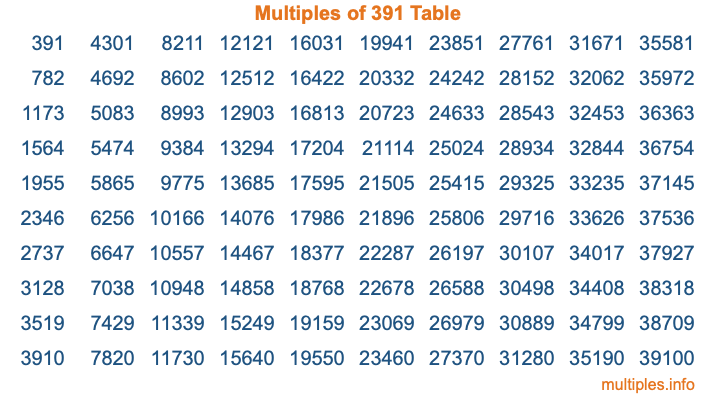Multiples of 391Welcome to the Multiples of 391 page. Here we will first teach you everything you will ever need to know about the multiples of 391, and then give you a study guide summary of everything we taught you to make sure you remember it all. Use this page to look up facts and learn information about the multiples of 391. This page will make you a multiples of three hundred ninety-one expert!

Definition of Multiples of 391
Multiples of 391 are all the numbers that when divided by 391 equal an integer. Each of the multiples of 391 are called a multiple. A multiple of 391 is created by multiplying 391 by an integer.

Therefore, to create a list of multiples of 391, you start with 1 multiplied by 391, then 2 multiplied by 391, then 3 multiplied by 391, and so on for as long as you want. Thus, the list of the first five multiples of 391 is 391, 782, 1173, 1564, and 1955. To see a larger list of multiples of 391, see the printable image of Multiples of 391 further down on this page. We also have a category where you can choose any nth multiple of 391.

Multiples of 391 Checker
The Multiples of 391 Checker below checks to see if any number of your choice is a multiple of 391. In other words, it checks to see if there is any number (integer) that when multiplied by 391 will equal your number. To do that, we divide your number by 391. If the the quotient is an integer, then your number is a multiple of 391.

Is  a multiple of 391?

Least Common Multiple of 391 and ...
A Least Common Multiple (LCM) is the lowest multiple that two or more numbers have in common. This is also called the smallest common multiple or lowest common multiple and is useful to know when you are adding our subtracting fractions. Enter one or more numbers below (391 is already entered) to find the LCM.

Check out our LCM Calculator if you need more details about the Least Common Multiple or if you need the LCM for different numbers for adding and subtraction fractions.

nth Multiple of 391
As we stated above, 391 is the first multiple of 391, 782 is the second multiple of 391, 1173 is the third multiple of 391, and so on. Enter a number below to find the nth multiple of 391.

th multiple of 391

Multiples of 391 vs Factors of 391
391 is a multiple of 391 and a factor of 391, but that is where the similarities end. All postive multiples of 391 are 391 or greater than 391. All positive factors of 391 are 391 or less than 391.

Below is the beginning list of multiples of 391 and the factors of 391 so you can compare:

Multiples of 391: 391, 782, 1173, 1564, 1955, etc.

Factors of 391: 1, 17, 23, 391

As you can see, the multiples of 391 are all the numbers that you can divide by 391 to get a whole number. The factors of 391, on the other hand, are all the whole numbers that you can multiply by another whole number to get 391.

It's also interesting to note that if a number (x) is a factor of 391, then 391 will also be a multiple of that number (x).

Multiples of 391 vs Divisors of 391
The divisors of 391 are all the integers that 391 can be divided by evenly. Below is a list of the divisors of 391.

Divisors of 391: 1, 17, 23, 391

The interesting thing to note here is that if you take any multiple of 391 and divide it by a divisor of 391, you will see that the quotient is an integer.

Multiples of 391 Table
Below is an image of the first 100 multiples of 391 in a table. The table is in chronological order, column by column. The first column has the first ten multiples of 391, the second column has the next ten multiples of 391, and so on.The Multiples of 391 Table is also referred to as the 391 Times Table or Times Table of 391. You are welcome to print out our table for your studies.

Negative Multiples of 391
Although not often discussed or needed in math, it is worth mentioning that you can make a list of negative multiples of 391 by multiplying 391 by -1, then by -2, then by -3, and so on, to get the following list of negative multiples of 391:

-391, -782, -1173, -1564, -1955, etc.

Multiples of 391 Summary
Below is a summary of important Multiples of 391 facts that we have discussed on this page. To retain the knowledge on this page, we recommend that you read through the summary and explain to yourself or a study partner why they hold true.

There are an infinite number of multiples of 391.

A multiple of 391 divided by 391 will equal a whole number.

391 divided by a factor of 391 equals a divisor of 391.

The nth multiple of 391 is n times 391.

The largest factor of 391 is equal to the first positive multiple of 391.

391 is a multiple of every factor of 391.

391 is a multiple of 391.

A multiple of 391 divided by a divisor of 391 equals an integer.

391 divided by a divisor of 391 equals a factor of 391.

Any integer times 391 will equal a multiple of 391.

Multiples of a Number
Here you can get the multiples of another number, all with the same attention to detail as we did for multiples of 391 on this page.

Multiples of
Multiples of 392
Did you find our page about multiples of three hundred ninety-one educational? Do you want more knowledge? Check out the multiples of the next number on our list!